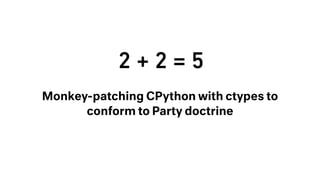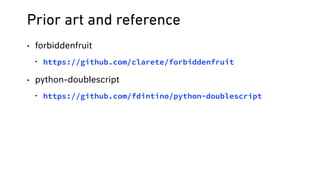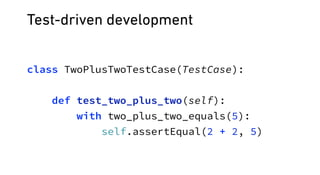Successfully reported this slideshow.×
1 of 34

2 + 2 = 5: Monkey-patching CPython with ctypes to conform to Party doctrine

1

Share

A few weeks into your tenure as a software engineer at the Ministry of Truth you are assigned your first real feature request: write a context manager that can make “2 + 2” equal 5 at runtime. Your solution should be written only in Python (for maximum portability). Absurd? Perhaps, but you know better than to ask questions. You are no thought-criminal.

In this talk I walk through the steps I took to modify the value of two plus two in CPython at runtime—using only Python and the ctypes module. What began for me as a silly and frivolous side project became an education in how the python data model works behind the scenes and how CPython compiles, optimizes, and executes python code.

The goal of this talk is to provide an introduction to CPython internals while walking through the steps needed to monkeypatch integer addition to make “2 + 2” equal 5. The audience should come away with a better understanding of how python objects and types are represented in memory, how references are counted, and how python scripts are transformed into abstract syntax trees, compiled into code objects, and then executed by the CPython virtual stack machine. And because I’ve limited myself to using ctypes, these topics can be explored without familiarity with C as a prerequisite.

More Related Content

See all

See all

2 + 2 = 5: Monkey-patching CPython with ctypes to conform to Party doctrine

1. 1. 2 + 2 = 5 Monkey-patching CPython with ctypes to conform to Party doctrine
2. 2. Prior art and reference • forbiddenfruit • https://github.com/clarete/forbiddenfruit • python-doublescript • https://github.com/fdintino/python-doublescript
3. 3. Test-driven development class TwoPlusTwoTestCase(TestCase):    def test_two_plus_two(self):  with two_plus_two_equals(5):  self.assertEqual(2 + 2, 5)
5. 5. ctypes crash course from ctypes import ( pythonapi, Structure, c_char_p, CFUNCTYPE)
6. 6. ctypes.pythonapi >>> from ctypes import pythonapi, c_char_p    >>> pythonapi.Py_GetVersion.restype = c_char_p  >>> pythonapi.Py_GetVersion()    2.7.13 (default, Feb 23 2017, 08:50:00) [GCC 4.2.1 Compatible Apple LLVM 8.0.0 (clang-800.0.42.1)]
7. 7. ctypes.py_object from ctypes import pythonapi, py_object    PyNumber_Absolute = pythonapi.PyNumber_Absolute  PyNumber_Absolute.argtypes = [py_object]  PyNumber_Absolute.restype = py_object    PyNumber_Absolute(-3) # 3
8. 8. ctypes.Structure class PyObject(Structure):  _fields_ = [  ('ob_refcnt', Py_ssize_t),  ('ob_type', py_object),  ]
9. 9. _CData.from_address(), POINTER class PyObject(Structure):  _fields_ = [  ('ob_refcnt', Py_ssize_t),  ('ob_type', py_object)]    py_object_p = ctypes.POINTER(py_object) # We will use this later foo = "foo"    pyobj = PyObject.from_address(id(foo))    print(pyobj.ob_refcnt) # 7  print(sys.getrefcount(foo)) # 8
10. 10. Overriding int.__add__ >>> print type(int.__dict__) <type 'dictproxy'> # Python 3 >>> print(type(int.__dict__)) <class 'mappingproxy'>
11. 11. Overriding int.__add__ typedef struct {  PyObject_HEAD  PyObject *dict;  } proxyobject;
12. 12. Overriding int.__add__ class DictProxy(PyObject):  _fields_ = [  ('dict', ctypes.POINTER(PyObject)),  ]
13. 13. Overriding int.__add__ def mutable_class_dict(cls):  dp = DictProxy.from_address(id(cls.__dict__))  temp = {}  pythonapi.PyDict_SetItem(  py_object(temp),  py_object(None),  dp.dict)  return temp[None]
15. 15. Overriding int.__add__ >>> 2 + 2  4   >>> (2).__add__(2)  5
16. 16. Why doesn’t overriding __add__ suffice? PyObject *  PyNumber_Add(PyObject *v, PyObject *w)  {  PyObject *result = binary_op1(v, w, NB_SLOT(nb_add));  if (result == Py_NotImplemented) { /* ... */ }  return result;  }
17. 17. /* object.h */ typedef struct _typeobject { PyObject_VAR_HEAD  const char *tp_name; /* For printing, in format "<module>.<name>" */  Py_ssize_t tp_basicsize, tp_itemsize; /* For allocation */    /* Methods to implement standard operations */  destructor tp_dealloc;  printfunc tp_print;  getattrfunc tp_getattr;  /* ... */    /* Method suites for standard classes */  PySequenceMethods *tp_as_sequence;  PyMappingMethods *tp_as_mapping;    /* ... */  } PyTypeObject; PyNumberMethods *tp_as_number;
18. 18. /* object.h */ typedef PyObject * (*binaryfunc)(PyObject *, PyObject *); typedef struct {  binaryfunc nb_add;  binaryfunc nb_subtract;  binaryfunc nb_multiply;  /* ... */  } PyNumberMethods; • binaryfunc: a pointer to a function that takes two PyObject pointers as arguments and returns a pointer to a PyObject • Use ctypes.CFUNCTYPE(return_type, *arg_types) binaryfunc = ctypes.CFUNCTYPE(py_object_p, py_object_p, py_object_p) typedef PyObject * (*binaryfunc)(PyObject *, PyObject *);
19. 19. /* object.h */ typedef PyObject * (*binaryfunc)(PyObject *, PyObject *); typedef struct {  binaryfunc nb_add;  binaryfunc nb_subtract;  binaryfunc nb_multiply;  /* ... */  } PyNumberMethods; • Use ctypes.Structure to represent the PyNumberMethods struct: class PyNumberMethods(Structure):  _fields_ = [  ('nb_add', binaryfunc),  ('nb_subtract', binaryfunc),  ('nb_multiply', binaryfunc),  # ...  ] typedef struct {  binaryfunc nb_add;  binaryfunc nb_subtract;  binaryfunc nb_multiply;  /* ... */  } PyNumberMethods;
20. 20. class PyTypeObject(PyObject):  _fields_ = [  ('ob_size', Py_ssize_t),  ('tp_name', c_char_p),  ('tp_basicsize', Py_ssize_t),  ('tp_itemsize', Py_ssize_t),  ('...', c_void_p * 6), # skip 6 functions, like tp_repr, for brevity  ('tp_as_number', POINTER(PyNumberMethods)), # ...  ]  PyInt_Type = PyTypeObject.from_address(id(int)) >>> PyInt_Type.tp_as_number.contents.nb_add(2, 2)  4